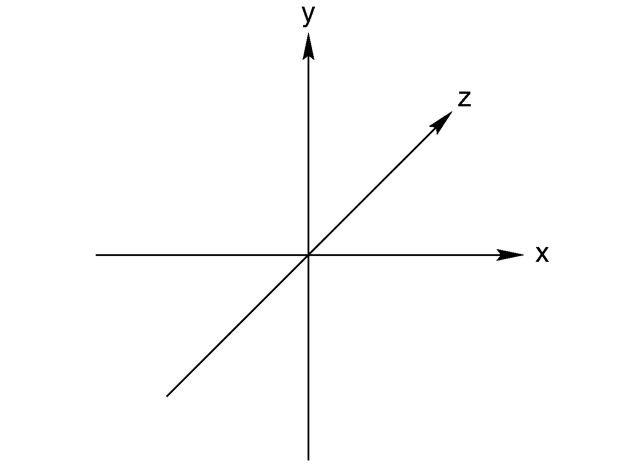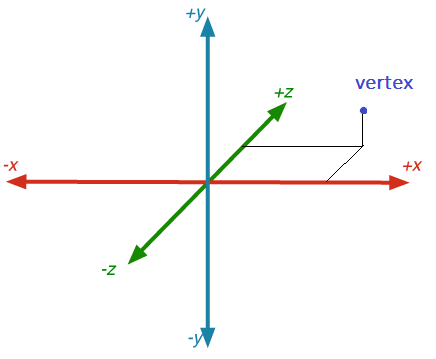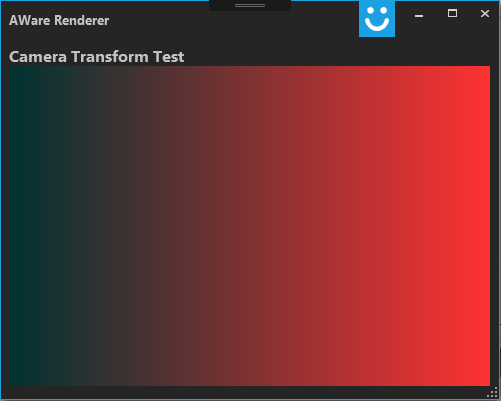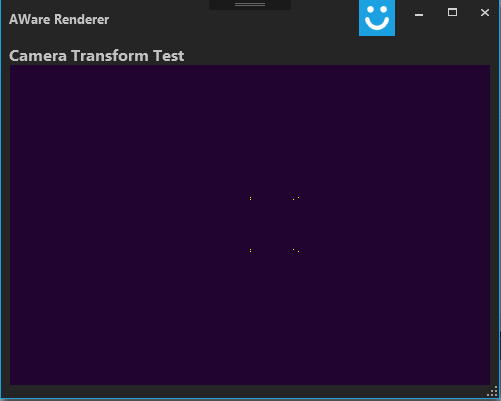# GPU Project 03 – World and space

So, now that we have a way of showing images, let start digging into how we will actually generate the images that we will show. The basic idea is that a 3D scene is formed by a digital representation of the objects that we want to show, and that a video card transforms this information into a 2d image that we can see on a screen. The first side is usually the task of really 3d designers, game creators, artists and whismy programmers that create collections of points, lights and textures that represent a 3d object. Since we are starting from scratch, we will start by trying to render a collection of points in space. These points will simply be a set of (x,y,z) points in the world. I always like to use coordinates the way PC game designers use them:This way the x and y coordinates make sense when you look at them in a monitor. X is the horizontal direction, y is the vertical direction and z is the depth looking into the monitor. So a vertex is determined by its coordinates in all three axis:So, let’s create a scene with a cube in it. All 8 vertexes would be located at these coordinates:

```cube[] = {
{0,0,0},
{1,0,0},
{0,1,0},
{1,1,0},
{0,0,1},
{1,0,1},
{0,1,1},
{1,1,1},
}
```

Now. How do we make these this collection of points appear on a 2d image? This problem has been well thought and understood in current literature. First off we need to model the 3d world a bit. We will place a virtual spectator in the world. This spectator will be single point and will be an infinitesimal pupil that takes in the light rays from the scene. The pupil will see the projection of the objects that are in front of it like if it were a small screen. The location of each point in the screen can be determined by this equation:

``````bx = width  * camera_space.x * (fx / camera_space.z);
by = height * camera_space.y * (fy / camera_space.z);``````

Where camera_space. x, .y and .z are the coordinates of the point in the camera space reference (we will talk a bit more about this later on), and fx and fy determine the field of view in the x and y coordinates respectively.

These variables can be calculated like so:

``````fx = 1.0 / Tan(fov_x / 2);
fy = 1.0 / Tan(fov_y / 2);``````

Since I will be testing out multiple algorithms I wanted to make sure that the equations actually worked before committing the time to implementing them in HW. I created a WPF application in visual studio that allows me translate an array into a bitmap that I can see on my display. I created an 800×600 pixel bitmap image that I can programatically modify.

``````// Create byte array
byte[] imgdata = new byte[width * height * bytesperpixel];

// Convert framebuffer to bitmap
BitmapSource bitmap_i = BitmapSource.Create(width, height, 118, 118, PixelFormats.Bgra32, null, imgdata, stride);``````

Here is a ramp that I tried out:

``````for (int y = 0; y < height; y++)
{
for (int x = 0; x < width; x++)
{
drawPixel(x, y, x*255 / width, 50, 50);
}
}``````Here is the current code to render a frame. Note that I added in some classes (structures) to keep track of objects and the vertexes that each of them has:

``````public void renderFrame()
{
// FOV calculations
double fov_x = 90 * Math.PI / 180.0;
double fov_y = 60 * Math.PI / 180.0;
double fx, fy;
fx = 1.0f / Math.Tan(fov_x / 2);
fy = 1.0f / Math.Tan(fov_y / 2);

foreach (Object obj in scene)
{
foreach( Point vertex in obj.vertexes)
{
double bx, by;
Point camera_space;

camera_space = vertex;
bx = width * camera_space.x * (fx / camera_space.z);
by = height * camera_space.y * (fy / camera_space.z);
drawPixel((int)bx + (width/2), (height/2-1)-(int)by, 255, 250, 0);
}
}
}``````

Running this code with the list of vertexes that I mentioned above produces this:So, it might not be the most impressive cube, but it looks like the projection is working! Next week we’ll try to experiment with rendering more complicated objects and placing the cube in different locations. Once we have those equations worked out we’ll switch over to RTL and start figuring out how to implement it in HW.